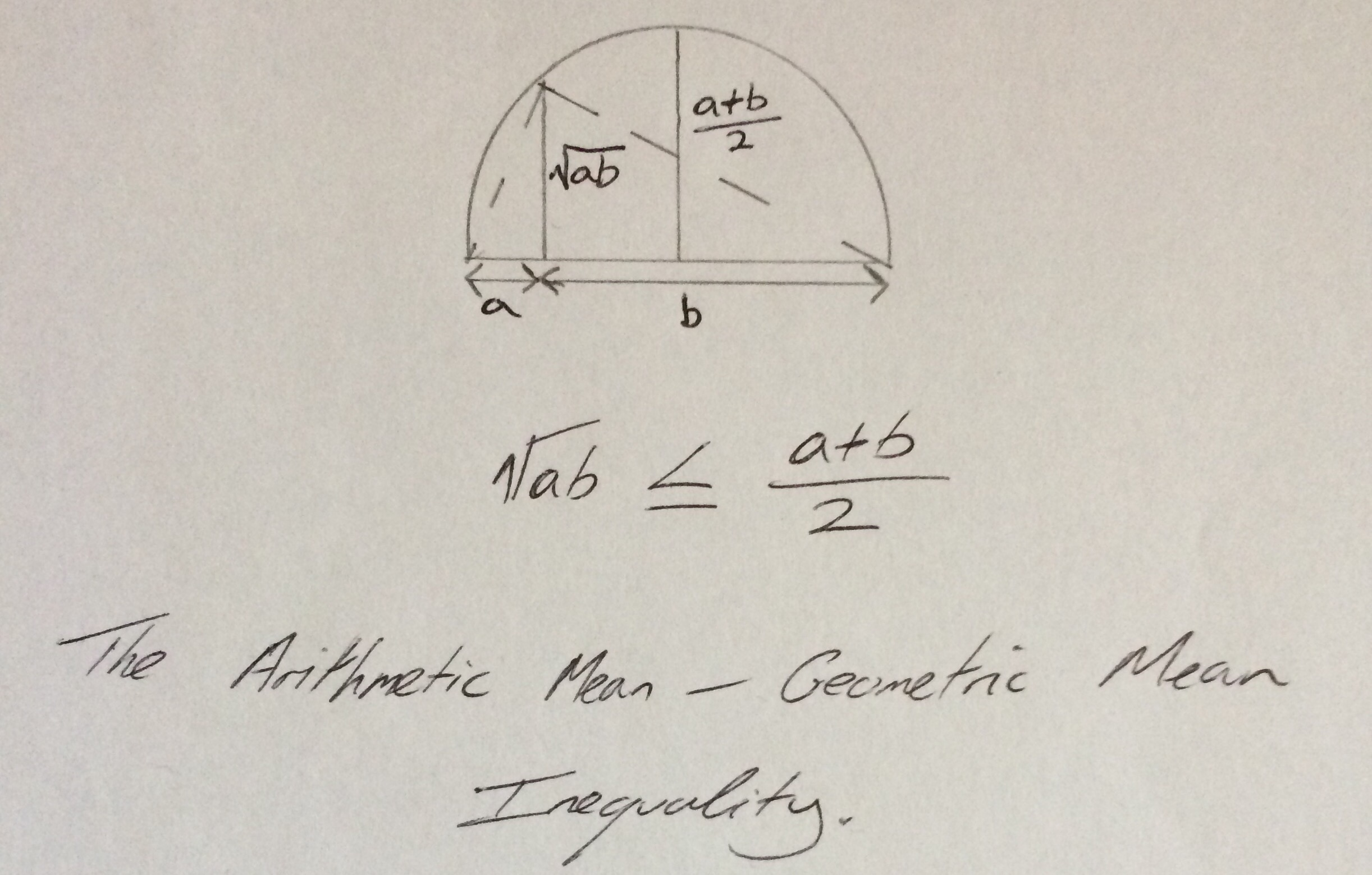Categories

# AM-GM Inequality

The Arithmetic Mean – Geometric Mean (AM-GM) inequality is possibly one of my favourite inequalities (another is the Cauchy-Schwarz inequality, see a later #summerblogchallenge post for more details on this).

Earlier this week I was honoured to have received an envelope of goodies from Ed (@solvemymaths). Alongside the very cool mathematical Mr Men stickers, there was a note from Ed with a lovely geometric illustration of the AM-GM for two lengths $$a,b$$.In words, the AM-GM inequality states that the arithmetic mean of a set of (non-negative) numbers is always greater than or equal to the geometric mean of the set of numbers. More formally, the AM-GM inequality can be stated as follows: For a set of non-negative real numbers $$a_1,a_2,a_3,…,a_n$$ the following inequality holds

$$\frac{a_{1}+a_{2}+a_{3}+ \cdots + a_{n}}{n} \geq \sqrt[n]{a_{1}a_{2}a_{3} \cdots a_{n}}$$

Example: For the set $$1,6,9,15$$ the arithmetic mean is $$\frac{31}{4} = 7.75$$ and the geometric mean is $$\sqrt{810} \approx 5.3348 [\latex]. Proof: There are many different proofs of the AM-GM inequality. The excellent Art of Problem Solving website lists a few here . I particularly like the one using the rearrangement inequality as it is so concise. The AM-GM inequality is often useful for questions on STEP and Maths Challenge papers, here is a typical question and a solution. Question: Given that [latex]a,b,c$$ are non-negative integers, show that

$$(a+b)(b+c)(a+c) \geq 8abc$$

Solution: Given that $$a,b$$ then applying the AM-GM inequality we have that

\begin{align} \frac{a+b}{2} &\geq \sqrt{ab} \\ a+b &\geq 2\sqrt{ab} \end{align}

Performing similar calculations with the other pairings we can obtain

\begin{align} b + c &\geq 2\sqrt{bc} \\ a+c &\geq \sqrt{ac} \end{align}

So, since the numbers involved are all positive we can multiply the inequalities to obtain

\begin{align} (a+b)(b+c)(a+c) &\geq 2\sqrt{ab}2\sqrt{bc}2\sqrt{ac} \\ &= 8\sqrt{abbcac} \\ &= 8\sqrt{a^2b^2c^2} \\ &=8abc \end{align}

as desired.

Can you come up with a nicer solution? Can you come up with similar questions?

The AM-GM inequality comes up in higher level maths too, a friend, Edward Hall of the University of Leicester has reminded me that it is used in a modified form when proving the continuous stability of the solutions to parabolic PDEs. He also mentioned that he has recently seen it used in a paper concerning Discontinuous Galerkin (more on these in the future) methods for quasilinear PDEs.